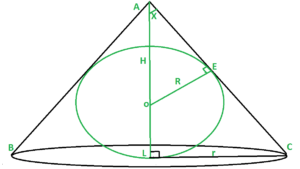# Minimum volume of cone that can be circumscribed about a sphere of radius R

• Last Updated : 07 Aug, 2022

Given a sphere of radius R, The task is to find out the minimum volume of the cone that can be circumscribed about it.Examples:

Input: R = 10
Output: Volume of cone = 8373.33
Explanation:
Radius of cone = 14.14 and Height of cone = 40,
Volume of cone =So, volume = 8373.33Input: R = 4 Output: Volume of cone = 535.89 

Approach:
we have given a sphere of radius R inscribed in Cone. We need to find out the radius and height of the cone to find out the volume of the cone.

1. In triangle AOE and ALC compute sin(X) i.e. For triangle AOEand for triangle ALC2. Now, From equating both we get3. Insert the value of H in Volume i.e.and for volume to be minimum4. From the above equation we getand putting this value in H we get5. Hence, applying the formula of volume of cone and puttingandwe get the desired result.

## C++

 // C++ program to find the minimum// volume of the cone that can be // circumscribed about a sphere// of radius R#includeusing namespace std; // Function to find the volume// of the conefloat Volume_of_cone(float R){         // r = radius of cone    // h = height of cone    // Volume of cone = (1 / 3) * (3.14) * (r*r) * (h)    // we get radius of cone from the derivation    // is root(2) times multiple of R    // we get height of cone from the derivation    // is 4 times multiple of R    float V = (1 / 3.0) * (3.14) * (2 * ( R * R ) ) * (4 * R);         return V;}     // Driver codeint main(){    float R = 10.0;    cout << Volume_of_cone(R);}     // This code is contributed by Samarth

## Java

 // Java program to find the minimum// volume of the cone that can be// circumscribed about a sphere// of radius Rimport java.util.*; class GFG{ // Function to find the volume// of the conestatic double Volume_of_cone(double R){         // r = radius of cone    // h = height of cone    // Volume of cone = (1 / 3) * (3.14) * (r*r) * (h)    // we get radius of cone from the derivation    // is root(2) times multiple of R    // we get height of cone from the derivation    // is 4 times multiple of R    double V = (double)((1 / 3.0) * (3.14) * (2 * (R * R)) *                                                  (4 * R));    return V;}     // Driver codepublic static void main(String[] args){    double R = 10.0;    System.out.print(Volume_of_cone(R));}} // This code is contributed by sapnasingh4991

## Python3

 # Python3 program to find the minimum# Volume of the cone that can be circumscribed# about a sphere of radius R import math # Function to find the volume# of the cone def Volume_of_cone(R):     # r = radius of cone    # h = height of cone    # Volume of cone = (1 / 3) * (3.14) * (r**2) * (h)    # we get radius of cone from the derivation    # is root(2) times multiple of R    # we get height of cone from the derivation    # is 4 times multiple of R         V = (1 / 3) * (3.14) * (2 * ( R**2 ) ) * (4 * R)         return V      # Driver codeif __name__ == "__main__":         R = 10         print(Volume_of_cone(R))

## C#

 // C# program to find the minimum// volume of the cone that can be// circumscribed about a sphere// of radius Rusing System;class GFG{ // Function to find the volume// of the conestatic double Volume_of_cone(double R){         // r = radius of cone    // h = height of cone    // Volume of cone = (1 / 3) * (3.14) * (r*r) * (h)    // we get radius of cone from the derivation    // is root(2) times multiple of R    // we get height of cone from the derivation    // is 4 times multiple of R    double V = (double)((1 / 3.0) * (3.14) *                    (2 * (R * R)) * (4 * R));    return V;}     // Driver codepublic static void Main(){    double R = 10.0;    Console.Write(Volume_of_cone(R));}} // This code is contributed by Nidhi_biet

## Javascript

 

Output:

8373.333333333332

Time complexity: O(1) since performing constant operations

Auxiliary space: O(1) since using constant variables

My Personal Notes arrow_drop_up